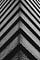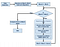# Automation package to impute missing values in a time series

## Introduction to ‘imputeTestbench’, an R packagePhoto by Callum Wale on Unsplash

# Background

Missing observations are common in time series data and several methods are available to impute these values prior to analysis. Variation in statistical characteristics of univariate time series can have a profound effect on the characteristics of missing observations and, therefore, the accuracy of different imputation methods.

The imputeTestbench package can be used to compare the prediction accuracy of different methods as related to the amount and type of missing data for a user-supplied dataset. Missing data are simulated by removing observations completely at random or in blocks of different sizes depending on the characteristics of the data. Several imputation algorithms are included with the package that varies from simple replacement with means to more complex interpolation methods. The testbench is not limited to the default functions and users can add or remove methods as needed. Plotting functions also allow comparative visualization of the behavior and effectiveness of different algorithms.

This post present example of applications that demonstrate how the package can be used to understand differences in prediction accuracy between methods as affected by characteristics of a dataset and nature of missing data.

# Missing Value Imputation

Identifying an appropriate imputation method is often the first step towards a more formal time series analysis. Different imputation methods will have differing precision in reproducing missing values, where precision will depend on how much data are missing and how the data are missing (i.e., individual observations missing at random or data missing in continuous chunks). The characteristics of the dataset will also influence imputation precision between methods.

An expectation is that imputation methods that leverage characteristics of the dataset to predict missing values will perform better than more naïve methods if indeed there is sufficient temporal structure. Accordingly, choosing an appropriate imputation method can be facilitated by using a standardized method of comparison.

A simple approach for method comparison is to evaluate prediction accuracy from imputed values after removing observations from a test dataset, where the test dataset should have characteristics similar to the one requiring imputation.

In such an approach, the imputation methods are compared by simulating different amounts of missing data, predicting the missing values with each method, and then comparing the predictions to the actual data that were removed. Then, the accuracy of predicted values is checked with statistical methods such as root-mean-squared error (RMSE) between observed and predicted data for each imputation method after removing and predicting 10% and 80% of the complete dataset.

In order to automate such a procedure, the imputeTestbench package is proposed, to simultaneously compare different imputation methods for univariate time series. The goal of this package is to provide an evaluation toolset that addresses the above challenges for identifying an appropriate imputation method before a more detailed analysis. This package provides several options for simulating missing observations with repeated sampling from a complete dataset. Missing values are imputed using any of several methods and then compared with a common error metric chosen by the user. Plotting functions are available to visualize the simulation methods for missing data, the predicted time series from each method, and the overall evaluation of prediction accuracy between methods.

Following is the theoretical foundation of imputeTestbench.The theoretical foundation of imputeTestbench

# Overview of imputeTestbench

Components of the workflow in the above figure are executed with the functions in imputeTestbench. The primary function is impute_errors() which is used to evaluate different imputation methods with missing data that are randomly generated from a complete dataset. The sample_dat() function is used to generate missing data within impute_errors() and includes a plotting option to demonstrate how the missing data are generated. The default error metrics for the imputed data are in the error_functions() function. The remaining two functions, plot_impute() and plot_errors(), are used to visualize imputation results and error summaries for the chosen methods.

## The impute_errors() function:

The impute_errors() function evaluates the accuracy of different imputation methods based on changes in the amount and type of missing observations from the complete dataset. The default methods included in impute_errors() are three methods for linear interpolation (na.approx(), zoo; na.interp(), forecast; na.interpolation(), imputeTS), last-observation carried forward (na.locf(), zoo), and mean replacement (na.mean(), imputeTS).

These methods are routinely applied in time series analysis, are easily understood compared to more complex approaches, and have relatively short computation times. Moreover, these methods represent a gradient from none to more complex dependence on the serial correlation of the time series — replacing missing data with overall means (na.mean()), replacing missing data with the last prior observation (na.locf()), and gap interpolation with linear methods (na.approx(), na.interp(), na.interpolation()). Note that the three linear methods vary considerably in the optional arguments that affect the imputation output. An expectation with the default methods included in imputeTestbench is varying imputation accuracy based on how each method relies on characteristics of a dataset to predict missing observations.

Although we acknowledge that the effectiveness of a chosen method depends on the data, the default techniques represent a broad range that is sufficient for most applications. As noted below, additional methods can be added as needed.

The impute_errors() function has the following arguments:

impute_errors(dataIn, smps = “mcar”, methods = c(“na.approx”,”na.interp”, “na.interpolation”, “na.locf”, “na.mean”), methodPath = NULL, errorParameter = “rmse”, errorPath = NULL, blck = 50, blckper = TRUE, missPercentFrom = 10, missPercentTo = 90, interval = 10, repetition = 10, addl_arg = NULL)

dataIn: A ts (stats) or a numeric object that will be evaluated. The input object is a complete dataset to evaluate by simulating missing data for performance evaluation and comparison of imputation methods.

smps: The desired type of sampling method for removing values from the complete time series provided by dataIn. Options are smps = ‘mcar’ for missing completely at random (MCAR, default) and smps = ‘mar’ for missing at random (MAR). Both methods provide different approaches to generating missing data in time series. In general, MCAR removes individual observations where the likelihood of a single observation being removed does not depend on whether observations closer in time have also been removed. By contrast, MAR removes observations in continuous blocks such that the likelihood of an observation being removed depends on whether observations closer in time have also been removed. The methods are described in detail in the section Sampling methods for missing observations.

methods: Methods that are used to impute the missing values generated by smps: replace with means (na.mean()), last-observation carried forward (na.locf()), and three methods of linear interpolation (na.approx(), na.interp(), na.interpolation()). Additional arguments passed to each method can be included in addl_arg described below.

methodPath: A character string for the path of the user-supplied script that includes one to many functions passed to methods. The path can be absolute or relative within the current working directory for the R session. The impute_errors() function sources the file indicated by methodPath to add the user-supplied function to the global environment.

errorParameter: The error metric used to compare the true, observed values from dataIn with the imputed values. Metrics included with imputeTestbench are root-mean-squared error (RMSE), mean absolute percent error (MAPE), and mean absolute error (MAE). The metric can be changed using errorParameter = ‘rmse’ (default), ‘mape’, or ‘mae’.

errorPath: A character string for the path of the user-supplied script that includes one to many error methods passed to errorParameter.

blck: The block size for missing data if the sampling method is at random, smps = ‘mar’. The block size can be specified as a percentage of the total amount of missing observations to remove or as a number of time steps in the input dataset.

blckper: A logical value indicating if the value for blck is a percentage (blckper = TRUE) of the total number of observations to remove or a sequential number of time steps (blckper = FALSE) to remove for each block. This argument only applies if smps = ‘mar’.

missPercentFrom, missPercentTo: The minimum and maximum percentages of missing values, respectively, that are introduced in dataIn. Appropriate values for these arguments are 10 to 90, indicating a range from few missing observations to almost completely absent observations.

interval: The interval of missing data from missPercentFrom to missPercentTo. The default value is 10% such that missing percentages in dataIn are evaluated from 10% to 90% at an interval of 10%, i.e., 10%, 20%, 30%, …, 90%. Combined, these arguments are identical to seq(from = 10,to = 90,by = 10).

repetition: The number of repetitions at each interval. Missing values are placed randomly in the original data such that multiple repetitions must be evaluated for a robust comparison of the imputation methods.

Considering the default values for the above arguments, the impute_errors() function returns an “errprof” object as the error profile for the imputation methods:

The “errprof” object is a list with seven elements. The first element, Parameter, is a character string of the error metric used for comparing imputation methods. The second element, MissingPercent, is a numeric vector of the missing percentages that were evaluated in the input dataset. The remaining five elements show the average error for each imputation method at each interval of missing data in MissingPercent. The averages at each interval are based on the repetitions specified in the initial call to impute_errors(), where the default is repetition = 10. Although the print method for the “errprof” object returns a list, the object stores the unique error estimates for every imputation method, repetition, and missing data interval. These values are used to estimate the averages in the printed output and to plot the distribution of errors with plot_errors() shown below. All error values can be accessed from the errall attribute, i.e., attr(a,’errall’).

## The plot_impute() function

The third plotting function available in imputeTestbench is plot_impute(). This function returns a plot of the imputed values for each imputation method in impute_errors() for one repetition of sampling for missing data. The plot shows the results in individual panels for each method with the points colored as retained or imputed (i.e., original data not removed and imputed data that were removed). An optional argument, showmiss, can be used to plot the original values as open circles that were removed from the data. The plot_impute() function shows results for only one simulation and missing data type (e.g., smps = ‘mcar’ and b = 50). Although the plot from plot_errors() is a more accurate representation of the overall performance of each method, plot_impute() is useful to better understand how the methods predict values for a sample dataset.

Further, the impute_errors() function uses sample_dat() to remove observations for imputation from the input dataset. Observations are removed using one of two methods relevant for univariate time series: MCAR and MAR as shown in the following figure. Such further details are available in this article.

# Concluding Remarks

The packages such as imputeTestbench and ForecastTB assists the researchers or analysts in automating the comparative analysis and performance evaluation with simple 1–2 lines of codes in no time. Also, these packages provided excellent functions to visualize the comparison results. Sometimes, these analyses might not be the final report for a project, but it provides intuitive sights to the researchers to show in which direction the project will move. The comments from readers are most welcome. The further details of imputeTestbench are available here.

For any further details, feel free to comment on this post.

Author:

Dr. Neeraj Dhanraj Bokde,

Postdoctoral Researcher,

Aarhus University, Denmark

https://www.researchgate.net/profile/Neeraj_Bokde

Researcher in Data Sciences

## More from Neeraj Dhanraj

Researcher in Data Sciences